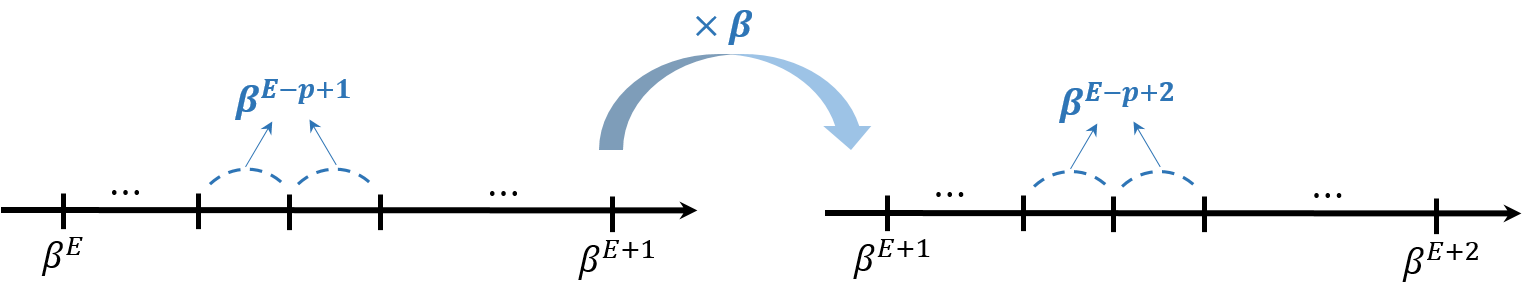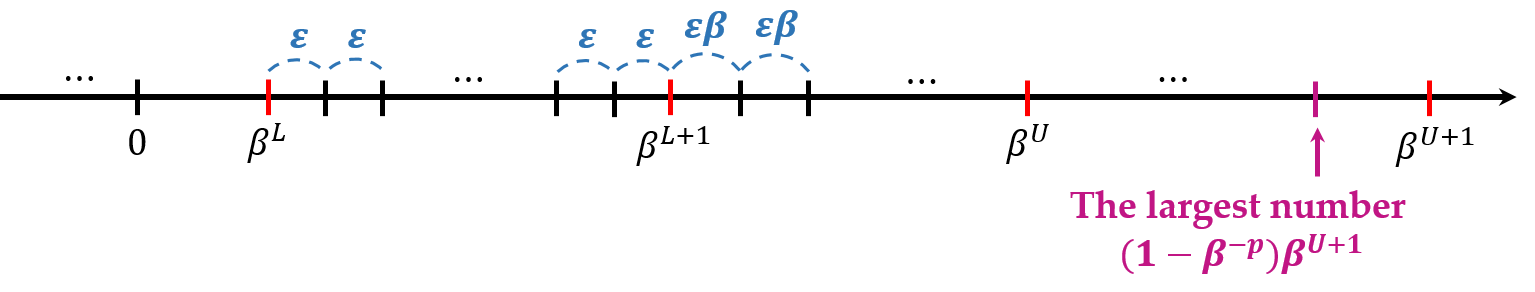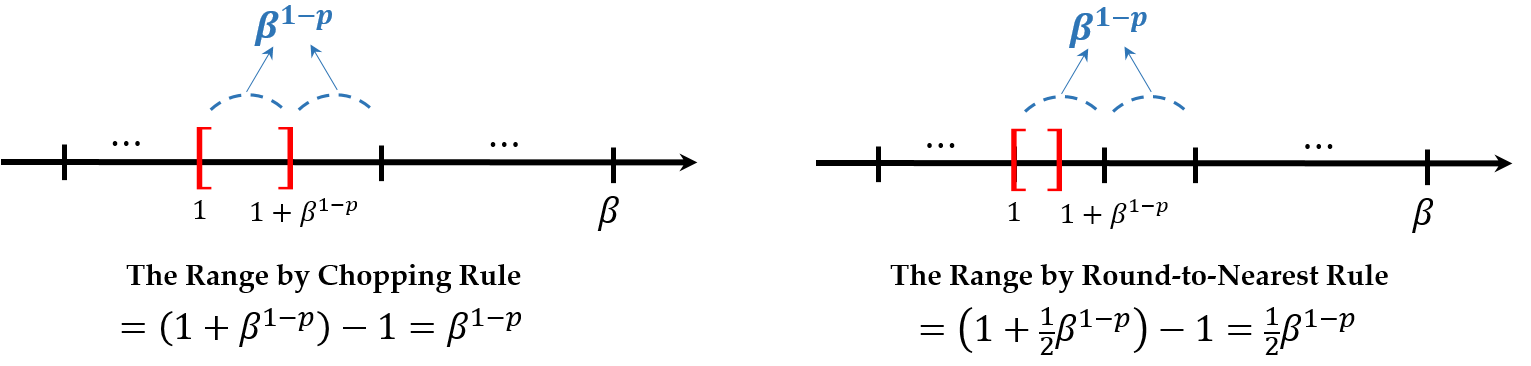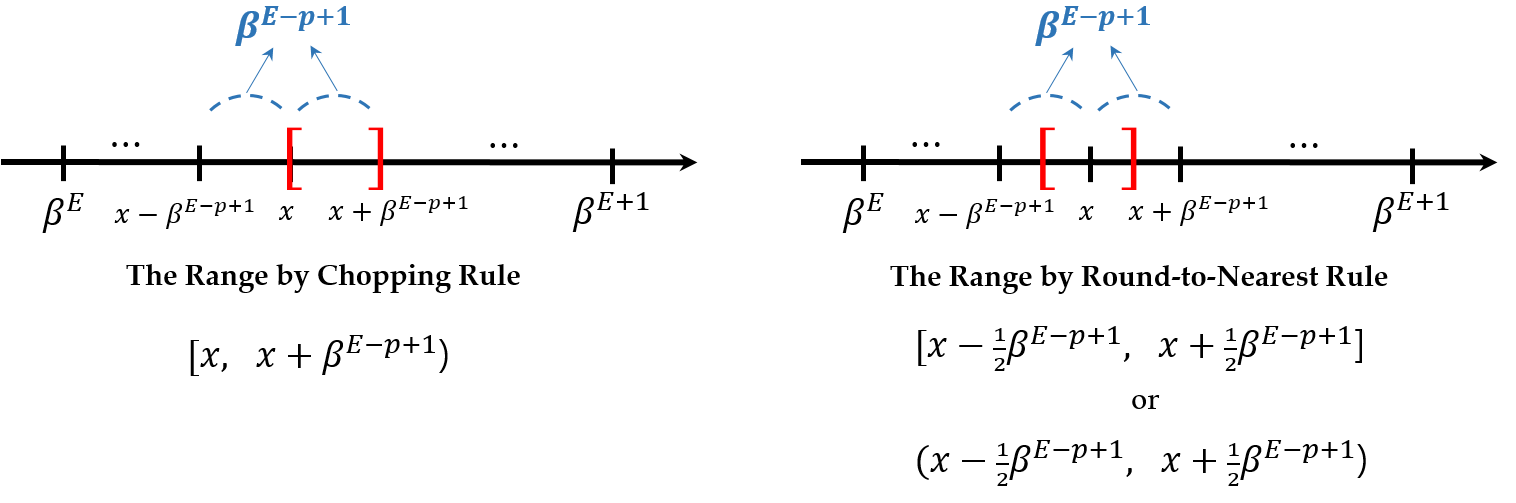# Floating-Point Number

## 1. System Format

Suppose that $\beta$ is the radix, or base, $p$ is precision, and $[L, U]$ is the range of exponent $E$. Then for $x \in \mathbb{R}$, \begin{aligned} x = \pm (d_0 . d_1 d_2 \mathellipsis d_{p-1})_{\beta} \beta^E = \pm \left( d_0 + \frac{d_1}{\beta} + \frac{d_2}{\beta^2} + \cdots + \frac{d_{p-1}}{\beta^{p-1}} \right) \beta^E \end{aligned}

where $d_i$ is an integer in $[0, \beta - 1]$.

• $p$-digit number based-$\beta$ $d_0 d_1 \mathellipsis d_{p-1}$: mantissa, or significant
• $d_1 \mathellipsis d_{p-1}$ of mantissa: fraction
• $E$: exponent, or characteristic

## 2. Normalization

For $x \not = 0 \in \mathbb{R}$, it can be normalized so that $d_0 \not = 0$ and mantissa $m$ is in $[1, \beta)$. This normalization is unique and saves space for leading zeros. Especially, $d_1$ is always $1$ when $\beta = 2$, so it does not have to ve stored and saves, in turn, one bit more.

• The number of the normalized floating-point number $x$ is
\begin{aligned} \underbrace{2}_{\pm} \times \underbrace{(\beta - 1)}_{d_0 \not = 0} \times \underbrace{(\beta^{p-1})}_{d_1 \thicksim d_{p-1}} \times \underbrace{(U - L + 1)}_{E} + \underbrace{1}_{\text{zero}} \end{aligned}
• The smallest positive $x$ is $(1.0\mathellipsis0)_{\beta} \beta^L = \beta^L$.
• The largest $x$ is
\begin{aligned} [(\beta - 1) . (\beta - 1) \mathellipsis (\beta - 1)]_{\beta} \beta^U &= (\beta - 1)(1 + \beta^{-1} + \cdots + \beta^{1-p}) \beta^U \\ &= \beta^{U+1} (1 - \beta^{-p}) \end{aligned}
• In general, floating point numbers are not uniformly distributed. However, they are uniformly distributed in the exponent range $[E, E+1)$ for $E \in \mathbb{Z}$. In this range, the minimal difference between numbers which floating-point system can represent is $(0.0\mathellipsis 1)_{\beta} \beta^E = \beta^{1-p} \beta^E = \beta^{E - p + 1}$. If this range is changed to $[E + 1, E + 2)$, then the minimal difference is multiplied by $\beta$.Let the minimal difference between numbers which floating-point system can represent in $[L, L + 1)$ be $\epsilon$. Then the following shows the entire distribution of floating-point numbers.The negative part is symmetrically the same as the positive one. Note that there could be the integers which the floating-point system cannot represent when this interval $\epsilon \beta^k > 1$.

## 3. Subnormal(Denormal) Numbers

When looking the series the floating-point system represents, there is empty space in $[0, \beta^L]$. This range can be divided by $\epsilon$, which is the interval in $[L, L + 1)$. Then the number in this range can be represented as $d_0 = 0$ and $d_1 \not = 0$, that is, $\pm (0. d_1 \mathellipsis d_{p-1})_{\beta}\beta^L$ if some condition are satisfied which will come later.

## 4. Rounding

The number which the floating-point system can exactly represent is called machine number. However, the number the system cannot do should be rounded. There are rules for rounding such as chopping or round-to-nearest method. Here are some examples about these rules when $p = 2$. \begin{aligned} \begin{array}{ccc} \text{number} & \text{chop} & \text{round-to-nearest} \\ 1.649 & 1.6 & 1.6 \\ 1.650 & 1.6 & 1.6 \\ 1.651 & 1.6 & 1.7 \\ 1.699 & 1.6 & 1.7 \end{array} \quad \begin{array}{ccc} \text{number} & \text{chop} & \text{round-to-nearest} \\ 1.749 & 1.7 & 1.7 \\ 1.750 & 1.7 & 1.8 \\ 1.751 & 1.7 & 1.8 \\ 1.799 & 1.7 & 1.8 \end{array} \end{aligned}

The round-to-nearest is also known as round-to-even, because it rounds the number to the one whose last digit is even in case of a tie. This rule is the most accurate and unbiased, but expensive. Meanwhile, IEEE standard system has the round-to-nearest as the default rule.

## 5. Machine Precision

The floating-point system can be measured by the machine precision, machine epsilon, or unit roundoff which is denoted by $\epsilon_{\text{mach}}$. It is the minimal number so that $1 + \epsilon_{\text{mach}} > 1$. Considering that the interval between the floating-point numbers in $[1, \beta)$ which can be exactly represented is $\beta^{1-p}$ because $E = 0$,$\epsilon_{\text{mach}} = \beta^{1-p}$ with rounding by chopping, and $\epsilon_{\text{mach}} = \frac{\beta^{1-p}}{2}$ with rounding-to-nearest. Now, consider the floating-point $x$ that can be exactly represented. Then there are many numbers that can be rounded to $x$.Therefore, the relative errors can be calculated as follows: \begin{aligned} \vert\text{relative error}\vert \le \begin{cases} \left\vert \frac{\beta^{E - p + 1}}{x} \right\vert = \frac{\beta^{E - p + 1}}{(d_0 . d_1 \mathellipsis d_{p-1}) \beta^E} \le \beta^{1-p} \quad \text{(chopping)} \\ \left\vert \frac{\frac{1}{2} \beta^{E - p + 1}}{x} \right\vert = \frac{\frac{1}{2} \beta^{E - p + 1}}{(d_0 . d_1 \mathellipsis d_{p-1}) \beta^E} \le \frac{1}{2} \beta^{1-p} \quad \text{(round-to-nearest)} \end{cases} \end{aligned}

It means that $\vert \text{relative error} \vert \le \epsilon_{\text{mach}}$.

## 6. IEEE Floating-Point Format

This system has $\beta = 2$, $p = 24$, $L = -126$, and $U = 127$ for $32$-bit floating-point numbers.Note that $d_0$ is always $1$ since $\beta = 2$, so $23$-bit mantissa can store only $23$-bit for $d_1 \mathellipsis d_{23}$ with $p = 24$. Its exponent is $8$-bit, so is in $[0, 255]$, but it is biased by $-127$. It yields that $L \le E - 127 \le U$, so $1 \le E \le 254$. Therefore, it can represent some special values when $E = 0$ or $E = 255$. \begin{aligned} \begin{cases} 1 \le E \le 254 \implies \pm (1. d_1 \mathellipsis d_{23})_2 2^{E-127} \quad \color{green}\text{normalized} \\ \\ E = 0 \quad \begin{cases} \text{mantissa} \not = 0 \implies \pm (0. d_1 \mathellipsis d_{23})_2 2^{-126} \quad \color{red}\text{subnormal} \\ \text{mantissa} = 0 \implies \pm 0 \end{cases} \\ \\ E = 255 \quad \begin{cases} \text{mantissa} \not = 0 \implies \text{NaN} \\ \text{mantissa} = 0 \implies \pm \infty \end{cases} \end{cases} \end{aligned}

• The smallest positive number is
\begin{aligned} \begin{cases} (1. 0 \mathellipsis 0)_2 2^{E-126} \approx 1.8 \times 10^{-38} \quad \color{green}\text{normalized} \\ \\ (0. 0 \mathellipsis 1)_2 2^{E-126} = 2^{-23} 2^{-126} \approx 1.4 \times 10^{-45} \quad \color{red}\text{subnormal} \end{cases} \end{aligned}
• The largest number is (1. 1 \mathellipsis 1)_2 2^{127} = (1 - 2^{-24}) 2^{128} \approx 3.4 \times 10^{38}.
• The machine epsilon $\epsilon_{\text{mach}}$ is
\begin{aligned} \epsilon_{\text{mach}} = \frac{1}{2} \beta^{1-p} = \frac{1}{2} 2^{1-24} = 2^{-24} \approx 10^{-7} \end{aligned}

since IEEE standard system uses the round-to-nearest as the default rounding rule. It has about $7$-precision in decimals. $\begin{gather} \log \epsilon_{\text{mach}} = \log 2^{-24} \approx -24 \times 0.3010 = -8 + \alpha, \quad \alpha \in [0, 1) \\ \implies \epsilon_{\text{mach}} = 2^{-24} = 10^{-8 + \alpha} < 10^{-7} \end{gather}$

## Reference

 Michael T. Heath, Scientific Computing: An Introductory Survey. 2nd Edition, McGraw-Hill Higher Education.